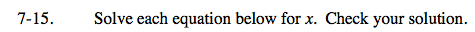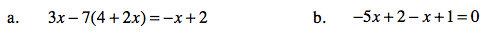### Home > AC > Chapter 7 > Lesson 7.1.2 > Problem7-15

7-15.
1. Solve each equation below for x. Check your solution. Homework Help ✎

1. 3x − 7(4 + 2x) = −x + 2

2. −5x + 2 − x + 1 = 0See problem 6-107.

x = −3